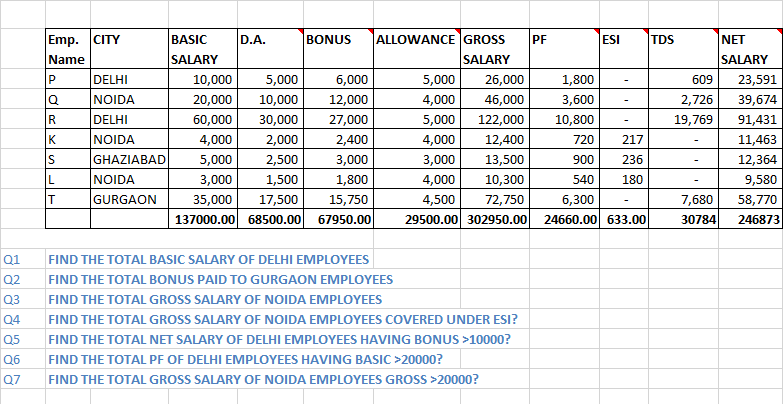Sumif and Sumifs

Accounts Tax
Excel

Resolve last question using Sumif and Sumifs FormulaQ1 FIND THE TOTAL BASIC SALARY OF DELHI EMPLOYEES

Q2 FIND THE TOTAL BONUS PAID TO GURGAON EMPLOYEES

Q3 FIND THE TOTAL GROSS SALARY OF NOIDA EMPLOYEES

Q4 FIND THE TOTAL GROSS SALARY OF NOIDA EMPLOYEES COVERED UNDER ESI?

Q5 FIND THE TOTAL NET SALARY OF DELHI EMPLOYEES HAVING BONUS >10000?

Q6 FIND THE TOTAL PF OF DELHI EMPLOYEES HAVING BASIC >20000?

Q7 FIND THE TOTAL GROSS SALARY OF NOIDA EMPLOYEES GROSS >20000?

#### Formula of Sumif

=sumif(Select range,"Write Criteria",Select Range whose sum required)

Note:-

If there are more than one criteria,we should use SUMIFS

#### Formula of Sumifs

=sumifs(Select Range whose sum required,Select range 1,"Write Criteria 1,Select range 2,"Write Criteria 2",)

Get live Maths 1-on-1 Classs - Class 6 to 12